Linear Transformations

What have linear systems got to do with Transformations?

We can think of a linear system as a transformation.
Some information is transformed into some other information.

Example: Buttons and Boxes

A shop keeper sells two different types of boxes of buttons.
 Box A contains 3 red buttons and 1 green button Box B contains 2 red buttons and 5 green buttons
Let
r=price of red buttons
g=price of green buttons
A=price of box A
B=price of box B
So, if the shop keeper wants r=2 cents, and g = 1 cent, he takes the information (r,g)=(2,1), and transforms it into the information (A,B).
A=3*r+1*g=6+1=7
B=2*r+5*g=4+5=9

He just tells you that (A,B)=(7,9), and you have to transform that information back into information about r and g, with the following linear algebra problem:
 3r + g = 7 2r + 5g = 9

But if the price of buttons changes, the shop keeper will still use the same packages, and still calculate the prices in the same way. But you'll get different prices for the boxes.
Eg. If red buttons go up in price to r=3, and green buttons go up in price to g=2, then
A=3*3+1*2=9+2=7
B=2*3+5*2=6+10=16

So we've transformed (3,2) to (11,16). We can represent this transformation on a graph: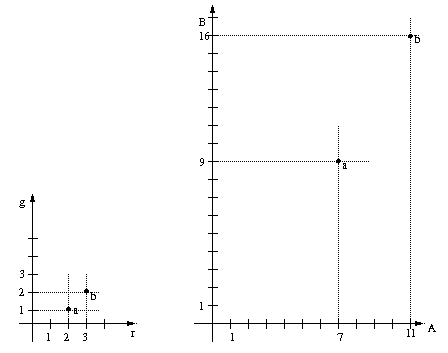Now, it might be that Mr Shop Keeper runs a button stock market, and different priced buttons are coming in and going out each day. So he wants a few more points on the graph, so he can easily look up prices.

Exercise

What are (A,B) when (r,g)=(1,1),(2,3),(2,2),(1,0),(0,1) or (0,0)?

Exercise

What are (r,g) if (A,B)=(5,12),(6,4),(3,15),(9,6) or (2,10)?
Calculate all the points, and plot them on the graphs, labeling corresponding points with the same number.
Now, is there a quick way to work out button box prices?
What you should have calculated is:
(r,g) -> (A,B)

And you should get a picture like this:-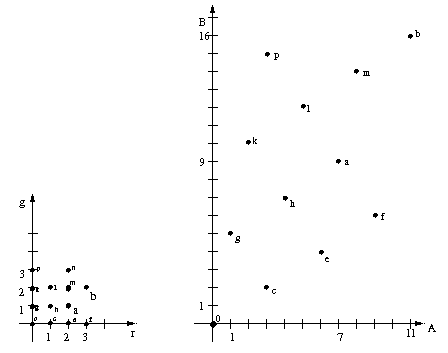Do you see the pattern?
Can you predict the image of (3,1), (1,3) and (3,3) just by looking at the graph? (ie, if (r,g)=(3,1) or (1,3) or (3,3), what is (A,B)?
It helps to draw some grid lines: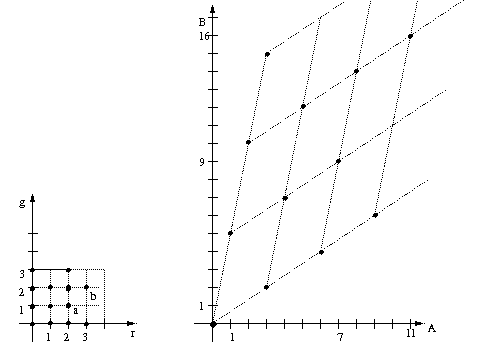The point is, the whole "grid" of points if transformed in a "linear" way, ie, straight lines stay straight lines, (0,0) stays at (0,0). (If the buttons are free, so are the boxes).
To find what the "image" of a point is (ie, where it gets transformed to), you just need to know where it is in the original grid, and then find the correponding point in the image. All you really need to know is "how far along? How far up?"
And the grid just depends on where (1,0) and (0,1) get transformed to. That's the points (3,2) and (1,5); they tell us all about what's going on.

What have can we do with Transformations?

We saw how a linear system can be viewed as a linear transformation.
Now we're going to look at linear transformations in more detail.
Linear maps preserve a lot of the structure of a graph, so they are pretty limited in some ways. For one thing, (0,0) must get mapped to (0,0), which is like having one point nailed to the floor.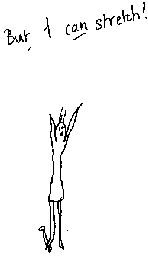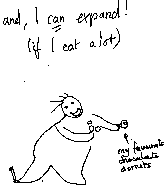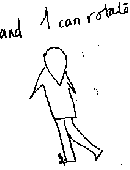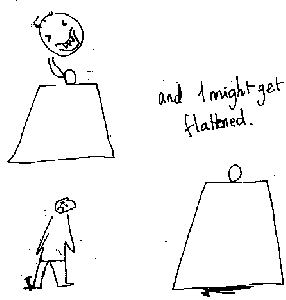But we can still do quite a lot with linear transformations.
eg:
• stretch
• expand
• rotate
• projection

Some examples of things that are linear maps:
(I might not be able to get pictures here, but I can show them in class with the OHP)
• Growth and form - some transformations in evolution in nature, due to D'arcy Thompson.
• Symmetries of platonic solids and others.

Some examples of things that are not linear maps:
• Growth and form - some transformations in evolution in nature, due to D'arcy Thompson.
• Wall paper transformation groups, and transformations with shifts.
• Inversion of circle, and mobius transformations.
• Transformations used to generate fractal sets.

(Note, some of the above can still be described by matrices, and vectors, and the tools of linear algebra are still very useful.)

Linear maps as symmetries

Another reason linear transformations are interesting is that we can think of them telling us about symmetries of things: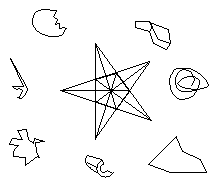(This picture is based on a much nicer one by Escher that I will show you in class.)
What is different about the object in the middle?
Well, it's "invariant" under quite a number of rotations.
We could think of these as linear transformations (the center of gravity stays fixed.)

Matrix Notation for Linear Maps

We really need to use some notation to describe these linear maps.
The first ones we looked at come from the linear system
 3r + g = A 2r + 5g = B

We tried all kinds of differnt values for (r,g), and got out different values for (A,B).
We found that the transformation depended on how the grid was transformed, and that depended on how the points (1,0) and (0,1) were transformed.
The new notation is: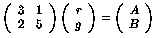This is a matrix equation.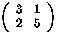is a matrix. (r,g) and (A,B) are vectors. (Well, if they are written like this, we can call them row vectors. In the equation, they appear as column vectors.)
A matrix can be viewed as just a notation used to write down a linear map. A vector can be viewed as a point on a graph. We'll talk more about the concepts of "matrix" and "vector" in the following lectures.
In the above equation, the matrix tells us what the transformation is, the vectors tell us what is being tranformed, and what it is being transformed into.

Important note

• The rows of the matrix come from the rows of the original equations for the transformation.
• The columns of the matrix are what the transformation does to (1,0) and (0,1).

Matrix and Vector Multiplication

Important Note

Although this little bit is very short, it is very important to remember the following definition.
In the above matrix equation,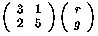means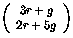Generally,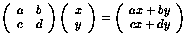What we are doing here is matrix and vector multiplication

Examples

Calculate the following: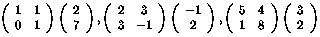Higher dimensional transformations, bigger matrices

Suppose we had three colours of buttons, instead of 2, and 3 different packs for sale.
eg:
 3 red + 2 green 1 yellow = Pack A 2 red + 1 green 4 yellow = Pack B 5 red + 2 green 2 yellow = Pack C

Then we'd be able to do exactly the same thing, and get a transformation of a three dimensional graph, and we can represent the transformation as a matrix, adn get a matrix and vector multplication. We will do this in class, and see how to multpliply matrices and vectors more generally.